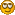# September Handicapping

Dawn
Battle Hill Farm

Total Days Handicapped: 9
Win: 40 x \$250 = \$10000
Place: 30 x \$100 = \$3000
Show: 25 x \$50 = \$1250
Quinella: 11 x \$500 = \$5500
Trifecta: 5 x \$1000 = \$5000
Total: \$24,750

Cyanne Black
Black Diamond Estates

Total Days Handicapped: 2
Wins: 8 x \$250 = \$2,000
Places: 6 x \$100 = \$600
Shows: 6 x \$50 = \$300
Trifecta: 1 x \$1000 = \$1,000
Quinella: – x \$500 = \$----
Total: \$3,900

Sarah
Blue Wolf Farm

Total Days Handicapped: 7
Win: 39 x \$250 = \$9,750
Place: 20 x \$100 = \$2,000
Show: 23 x \$50 = \$1,150
Quinella: 7 x \$500 = \$3,500
Trifecta: 3 x \$1000 = \$3,000
Total: \$19,400

Name: Nan
Stable: Lanson Farms

Days handicapped: 2
Total wins 7 x \$250 = \$1750
Total places 4 x \$100 = \$400
Total shows 6 x \$50 = \$300
Total exactas 3 x \$500 = \$1500
Total trifectas 1 x \$1000 = \$1000
Total money won: \$4950

Willow Brooke Stables
Lauren

Days Handicapped: 2
Win-      10  x  \$250    =  \$4000
Place-    5  x  \$100    =  \$500
Show-    3  x  \$50    =  \$150
Quinella-  7 x  \$500  =  \$3500
Trifecta-  6 x  \$1000  =  \$6000
Total-\$14,150

Bam
Wither Hawk Stables

Total Days Handicapped: 7
Wins: 24 x \$250 = \$6000
Places: 21 x \$100 = \$2100
Shows: 19 x \$50 = \$950
Trifecta: 3 x \$1000 = \$3000
Quinella: 10 x \$500 = \$5000
Total: \$17,450

Kiera
Dragonsden Stables

Total Days Handicapped: 4
14 wins x 250= 3500
8 places x 100= 800
12 shows x 50= 600
6 quinella x 500= 3000
0 trifecta x 1000= 0
Total=\$7900

Total days handicapped: 4
Wins: 18 X \$250 = \$4500
Places: 18 X \$100 = \$1800
Shows: 11 X \$50 = \$550
Trifecta: 0 X \$1000 = \$0
Quinella: 4 X 500 = \$2000
TOTAL: \$8850

Krystal
Menelya

Total Days Handicapped: 5
Win: 26 x \$250 = \$6500
Place: 12 x \$100 = \$1200
Show: 15 x \$50 = \$750
Quinella: 4 x \$500 = \$2000
Trifecta: 2 x \$1000 = \$ 2000
Total: \$12450

LeAnn
Freedom Acres

Total Days Handicapped: 2
Win: 3 x \$250 = \$750
Place: 9 x \$100 = \$900
Show: 7 x \$50 = \$350
Quinella: 2 x \$500 = \$1,000
Trifecta: 0 x \$1,000 = \$0
Total: \$3,000

I had to redo this because I realized I was hitting the Play-by-Play button when I checked my places.I hope this doesn’t screw anything up.

September Results

14 wins=3500
15 place=1500
12 show=600
4 exacta=2000
Total=\$7600

All the Sept. money has been awarded…sorry for the delay.

Thanks, Shanthi!